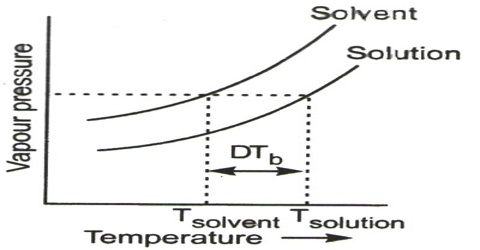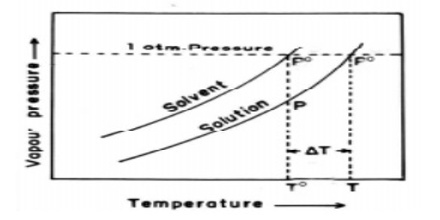# Elevation of Boiling Point

Elevation of Boiling Point

The boiling point of a liquid is defined as the temperature at which the vapour pressure is equal to the atmospheric pressure. As the vapour pressure of a solution is less than that of the solvent, the vapour pressure of the solution will become equal to the atmospheric pressure at a higher temperature. In other worth, the boiling point of the solution will be higher than that of the solvent. The vapour pressure-temperature diagrams of pure solvent and solution (Figure) clearly explain the elevation of boiling point of the solution compared with that of the pure solvent. So, Boiling point elevation is the incident that occurs when the boiling point of a liquid is increased when another amalgam is added, such that the solution has a higher boiling point than the pure solvent. Boiling point elevation occurs whenever a non-volatile solute is added to a pure solvent.Figure: vapour pressure vs temperature curves

The dotted horizontal line corresponds to 1.0 atm pressure. It intersects the vapour pressure curves at points corresponding to temperatures, T0 and T. It is seen that the boiling point, T, of the solution is higher (elevated) than the boiling point, T0, of the solvent. Thus the elevation of boiling point of a solution is a direct consequence of the lowering of vapour pressure on addition of solute.

Experimental results on the elevation of boiling point by solutes were summarized in the form of the following laws which are generally called Raoult’s laws of elevation of boiling point:

• The elevation of the boiling point of solution is proportional to its modal concentration, i.e., to its molality, m.
• Equimolecular quantities of different substances dissolved in the same quantity of a particular solvent raise its boiling point to the same extent.

Example: The boiling point of salted water is higher than the boiling point of pure water.